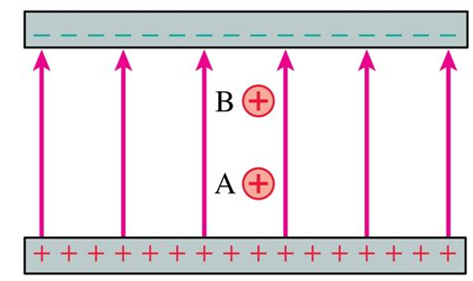# Problem: Two positive charges are equal. Which has more electric potential energy?a. Both have zero potential energyb. Charge Ac. Charge Bd. They have the same potential energy

###### FREE Expert Solution

A positive charge normally moves in the direction of the electric field.

When a charge moves in the direction it would move normally, its potential energy decreases.

96% (463 ratings)###### Problem Details

Two positive charges are equal. Which has more electric potential energy?a. Both have zero potential energy

b. Charge A

c. Charge B

d. They have the same potential energy